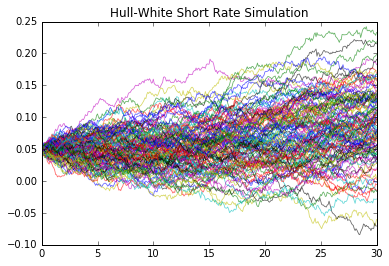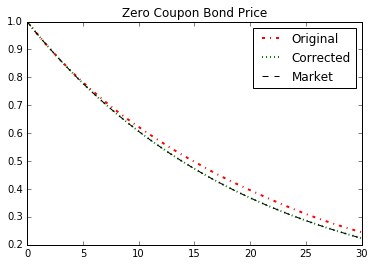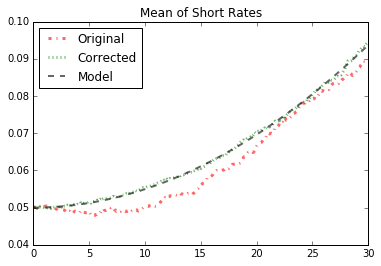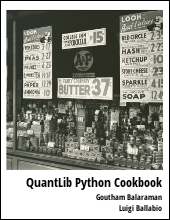This post explains how to use moment matching to reduce variance in Monte Carlo simulation of the Hull-White term structure model.

Visit here for other QuantLib Python examples. If you found these posts useful, please take a minute by providing some feedback.

In an earlier blog post on how the Hull-White Monte Carlo simulations are notorious for not coverging with some of the expected moments. In this post, I would like to touch upon a variance reduction technique called moment matching that can be employed to fix this issue of convergence.

The idea behind moment matching is rather simple. Lets consider the specific example of short rate model. For the short rate model, it is known that the average of stochastic discount factors generated from each path has to agree with the model (or the give yield curve) discount factors. The idea of moment matching is to correct the short rates generated by the term structure model such that the average of stochastic discount factors from the simulation matches the model discount factors.

In :
import QuantLib as ql
import numpy as np
import matplotlib.pyplot as plt
%matplotlib inline
from scipy.integrate import cumtrapz
ql.__version__

Out:
'1.11'

For simplicity, we use a constant forward rate as the given interest rate term structure. The method discussed here would work with any market yield curve as well.

In :
sigma = 0.01
a = 0.001
timestep = 360
length = 30 # in years
forward_rate = 0.05
day_count = ql.Thirty360()
todays_date = ql.Date(15, 1, 2015)

In :
ql.Settings.instance().evaluationDate = todays_date

yield_curve = ql.FlatForward(
todays_date,
ql.QuoteHandle(ql.SimpleQuote(forward_rate)),
day_count)
spot_curve_handle = ql.YieldTermStructureHandle(yield_curve)


Here, I setup the Monte Carlo simulation of the Hull-White process. The result of the generate_paths function below is the time grid and a matrix of short rates generated by the model. This is discussed in detaio in the Hull-White simulation post.

In :
hw_process = ql.HullWhiteProcess(spot_curve_handle, a, sigma)
rng = ql.GaussianRandomSequenceGenerator(
ql.UniformRandomSequenceGenerator(
timestep,
ql.UniformRandomGenerator(125)))
seq = ql.GaussianPathGenerator(hw_process, length, timestep, rng, False)

In :
def generate_paths(num_paths, timestep):
arr = np.zeros((num_paths, timestep+1))
for i in range(num_paths):
sample_path = seq.next()
path = sample_path.value()
time = [path.time(j) for j in range(len(path))]
value = [path[j] for j in range(len(path))]
arr[i, :] = np.array(value)
return np.array(time), arr


Here is a plot of the generated short rates.

In :
num_paths = 128
time, paths = generate_paths(num_paths, timestep)
for i in range(num_paths):
plt.plot(time, paths[i, :], lw=0.8, alpha=0.6)
plt.title("Hull-White Short Rate Simulation")
plt.show()The model zero coupon bond price $B(0, T)$ is given as: $$B(0, T) = E\left[\exp\left(-\int_0^T r(t)dt \right) \right]$$ where $r(t)$ is the short rate generated by the model. The expectation of the stochastic discount factor at time $T$ is the price of the zero coupon bond at that time. In a simulation the paths are generated in a time grid and the discretization introduces some error. The empirical estimation of the zero coupon bond price from a Monte Carlo simulation $\hat{B}(0, t_m)$ maturing at time $t_m$ is given as:

$$\hat{B}(0, t_m) = \frac{1}{N}\sum_{i=1}^{N} \exp\left(-\sum_{j=0}^{m-1} \hat{r}_i(t_j)[t_{j+1}-t_j] \right)$$

where $\hat{r}_i(t_j)$ is the short rate for the path $i$ at time $t_j$ on the time grid. The expression for the moment matched short rates is given as :

$$r^c_i(t_j) = \hat{r}_i(t_j) + \frac{\log \hat{B}(0, t_{j+1}) - \log \hat{B}(0, t_{j})}{t_{j+1} - t_j} - \frac{\log B(0, t_{j+1}) - \log B(0, t_{j})}{t_{j+1} - t_j}$$

In :
def stoch_df(paths, time):
return np.mean(
np.exp(-cumtrapz(paths, time, initial=0.)),axis=0
)
B_emp = stoch_df(paths, time)
logB_emp = np.log(B_emp)
B_yc = np.array([yield_curve.discount(t) for t in time])
logB_yc = np.log(B_yc)

In :
deltaT = time[1:] - time[:-1]
deltaB_emp = logB_emp[1:]-logB_emp[:-1]
deltaB_yc = logB_yc[1:] - logB_yc[:-1]

In :
new_paths = paths.copy()
new_paths[:,1:] += (deltaB_emp/deltaT - deltaB_yc/deltaT)


The plots below show the zero coupon bond price and mean of short rates with and without the moment matching.

In :
plt.plot(time,
stoch_df(paths, time),"r-.",
label="Original", lw=2)
plt.plot(time,
stoch_df(new_paths, time),"g:",
label="Corrected", lw=2)
plt.plot(time,B_yc, "k--",label="Market", lw=1)
plt.title("Zero Coupon Bond Price")
plt.legend()

Out:
<matplotlib.legend.Legend at 0x7f78aa2f1990>In :
def alpha(forward, sigma, a, t):
return forward + 0.5* np.power(sigma/a*(1.0 - np.exp(-a*t)), 2)

avg = [np.mean(paths[:, i]) for i in range(timestep+1)]
new_avg = [np.mean(new_paths[:, i]) for i in range(timestep+1)]
plt.plot(time, avg, "r-.", lw=3, alpha=0.6, label="Original")
plt.plot(time, new_avg, "g:", lw=3, alpha=0.6, label="Corrected")
plt.plot(time,alpha(forward_rate, sigma, a, time), "k--", lw=2, alpha=0.6, label="Model")
plt.title("Mean of Short Rates")
plt.legend(loc=0)

Out:
<matplotlib.legend.Legend at 0x7f78a8a3d2d0>### Reference

 Paul Glasserman, Monte Carlo Methods in Financial Engineering, Chapter 4

quantlib   python   finance

Related PostI am Goutham Balaraman, and I explore topics in quantitative finance, programming, and data science. You can follow me @gsbalaraman.

Checkout my bookUpdated posts from this blog and transcripts of Luigi's screencasts on YouTube is compiled into QuantLib Python Cookbook .